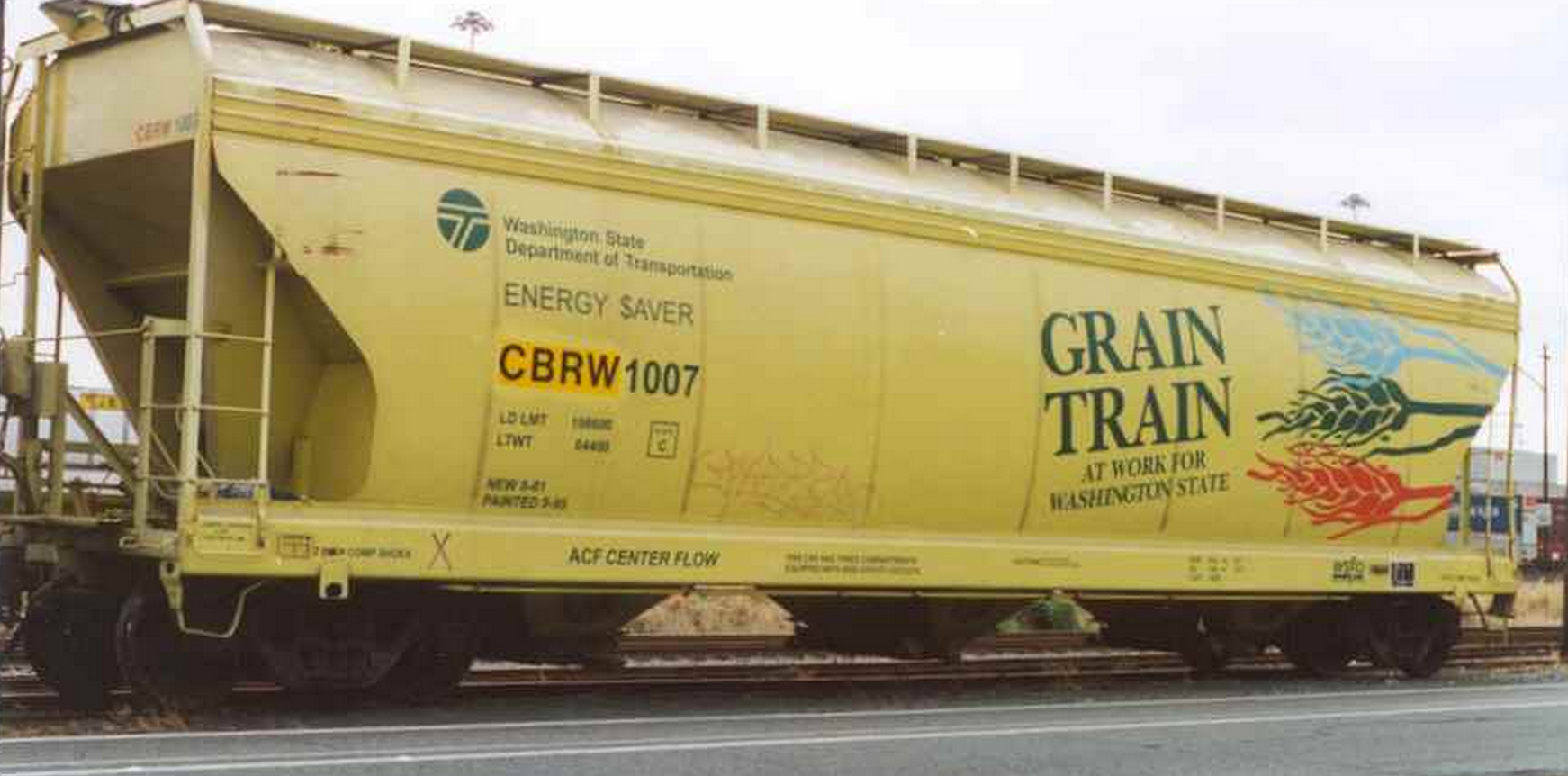# Coming in hot, coming out cold?Grain is loaded onto a train initially coasting at the speed $v_0 \text{ m/s}$ by dropping it vertically from a stationary silo at the rate $\alpha_m \text{ kg/s}$. How fast is the train moving when it exits from under the silo (in m/s)?

Details

• The train is $l = 200$ m long, and has an empty weight of $m_0 = 500$ kg.
• $v_0=45$ m/s
• $\alpha_m = 20$ kg/s
×